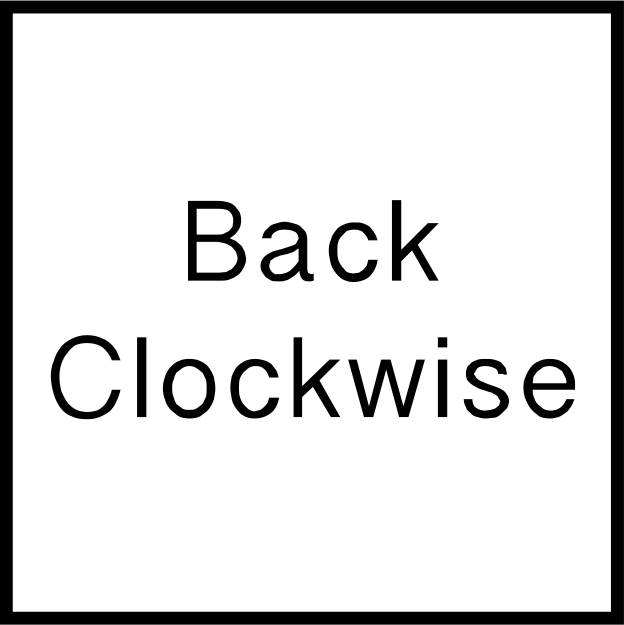# 3x3 Rubik's Cube Notation - How to read the lettersEver been watching a Rubik's cube tutorial video or reading a speed cubing article online, and come across that strange string of letters that somehow refer to moves on the cube? Here's a handy chart for basic 3x3 Rubik's Cube Notation.

## NotationTurn the Up (U) LayerTurn the Right (R) LayerTurn the Left (L) LayerTurn the Front (F) LayerTurn the Back (B) LayerTurn the Down (D) LayerTurn the middle (M) layer (Turns in the same direction as the L move)Turn the equator (E) layer (Turns the same direction as the D move)Turn the S layer (Turns the same direction as the F layer)

## Counterclockwise and 180°

All standard notation without any suffixes indicate a 90° clockwise rotation, e.g. R refers to a rotation of the right face 90° clockwise.

To indicate a 90° counterclockwise notation, an apostrophe ' is placed after the letter corresponding to the whichever face needs to be turned 90° counterclockwise,e.g. R' refers to a rotation of the right face 90° counterclockwise.

For a 180° rotation, a number 2 is placed after the corresponding letter similar to the apostrophe indicating counterclockwise moves, e.g. U2 refers to a rotation of the upper face 180° (counterclockwise/clockwise, dependent on case) - not the band!

## Wide Moves

Wide moves turn two layers at once and are indicated by either a lower case letter or a W suffix after a letter. For example, r or Rw refers to a 90° clockwise rotation of both the right and middle layers.## Brackets

In algorithm sites such as speedcubestats.com, you may see both square and/or round brackets containing letters. These brackets group like sets of turns together, commonly known as triggers.

For example, an algorithm for OLL (Orientation of the Last Layer) 20 features a R U R' trigger enclosed between circular brackets. A variant of this can be found in OLL 56, where a similar trigger is enclosed but wide R moves are used instead.

 OLL Diagram Algorithm 20 S' (R U R') S U' M' U R U' r' 56 (r U r') (U R U' R') (U R U' R') (r U' r')

Triggers are useful for breaking up the algorithms into "chunks" - which can make learning algorithms a lot easier and more digestable.

After having learned basic speed cubing notation, the next step is to start implementing more efficient fingertricks for turning the puzzle - check out J Perm's video tutorial on 3x3 fingertricks.

« Back to Blog

You have successfully subscribed!
This email has been registered